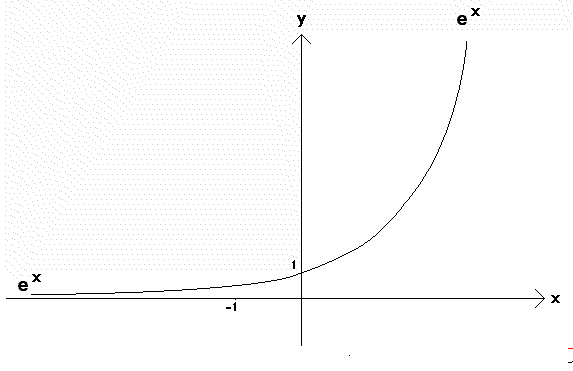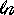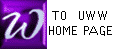Taylor Series
Taylor Polynomials

A Power series is a series (or formal sum) with the form : a0 + a1x1 + a2x2 + a3x3 + a4x4 + a5x5 + ....

A Taylor series about the base number 0 for a function f is a Power series in which the numbers " an " are chosen as follows:

an =
 f (n)(0)n !
= [ f (n)(0) ] ÷ n !

An animated example of Taylor Polynomials for the function f(x) = ex

The first 5 Taylor Polynomials (in red) for y = ex (in black) at base a = 0

For each value of x, Taylor polynomials become successively better approximations to ex as more terms are added.
Each Taylor Poynomial becomes a gradually poorer approximation to ex as x moves away from the base point a = 0

P0 = 1
P1 = 1 + x
P2 = 1 + x + (½)x2
P3 = 1 + x + (½)x2 + ()x3
P4 = 1 + x + (½)x2 + ()x3 + (1/24)x4An example of the calculation of the Taylor series

Here we create several terms of the Taylor series (about the base a=0 ) for f(x) =(1 - x)
Note: x must be less than 1 because (x ) is not defined for negative x

 Step 1: Calculate the derivatives of(1 - x)(1 - x) is a chain or composition: use Chain rule
u = 1 - x
 dudx = - 1
y(u) =u
 dydu = u- 1
Thus, the derivative of(1 - x) is the product of the above derivatives: u- 1 (- 1 ) = (- 1) (1 - x)- 1
Similarly, the derivative of (1 - x)- n will be: (- n ) (1 - x)- n - 1(- 1) = +n (1 - x)- n - 1 . Enter these derivatives in the table below: derivative #0 is the original function(1 - x)

 n function: f (n)(x) value at    x = 0 an 0(1 - x) 1 (- 1) (1 - x)- 1 2 (- 1) (1 - x)- 2 3 (- 1)(2) (1 - x)- 3 4 (- 1)(2)(3) (1 - x)- 4 5 (- 1)(2)(3)(4) (1 - x)- 5

 Step 2: Enter the values of the derivatives at x = 0 into the table above, as shown below

 n function: f (n)(x) f (n)(0) an 0(1 - x) 0 1 (- 1) (1 - x)- 1 - 1 2 (- 1) (1 - x)- 2 - 1 3 (- 1)(2) (1 - x)- 3 (- 1)(2) 4 (- 1)(2)(3) (1 - x)- 4 (- 1)(2)(3) 5 (- 1)(2)(3)(4) (1 - x)- 5 (- 1)(2)(3)(4)

 Step 3: Divide the numbers from Step 2 in the table above by n !, as shown in the right-most column below

 n function: f (n)(x) f (n)(0) an = [ f (n)(0) ] ÷ n ! 0(1 - x) 0 0 ÷ 0! = 0 ÷ 1 = 0 1 (- 1) (1 - x)- 1 - 1 (- 1) ÷ 1! = (- 1) ÷ 1 = (- 1) 2 (- 1) (1 - x)- 2 - 1 (- 1) ÷ 2! = (- 1) ÷ 2 = (- ½) 3 (- 1)(2) (1 - x)- 3 (- 1)(2) (- 1)(2) ÷ 3! = (- 1)(2) ÷ (3)(2) = (- ⅓) 4 (- 1)(2)(3) (1 - x)- 4 (- 1)(2)(3) (- 1)(2)(3) ÷ 4! = (- 1)(2)(3) ÷ (2)(3)(4) = (- ¼) 5 (- 1)(2)(3)(4) (1 - x)- 5 (- 1)(2)(3)(4) (- 1)(2)(3)(4) ÷ 5! = (- 1)(2)(3)(4) ÷ (2)(3)(4)(5) = (- ⅕)

The Taylor series for f(x) =(1 - x) is therefore :
 0 + (- 1)x + (- ½)x2 + (- ⅓)x3 + (- ¼)x4 + (- ⅕)x5 +....